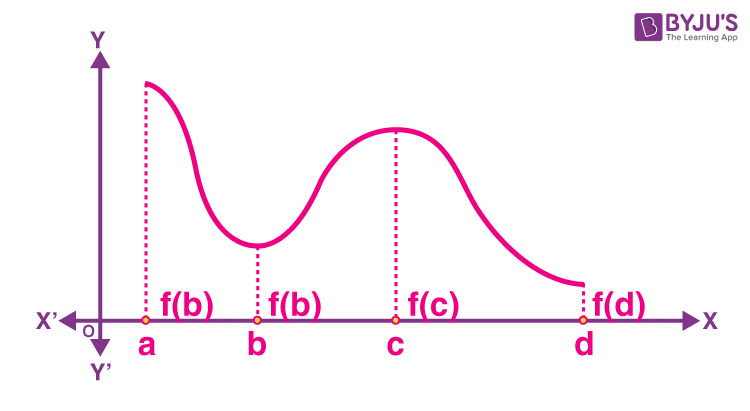# Maximum and Minimum Values of a Function in a Closed Interval

In calculus, you might have learnt how to find the local maximum and local minimum values of a function using the first derivative test and second derivative test. As we know, the function has neither a local maximum value nor a local minimum value. In this article, you will learn how to find the minimum and maximum values of a function in a closed interval in detail.

Let us consider a function f(x) = x + 3 such that x ∈ (0, 1).

Observe that the function is continuous on (0, 1), and thus, the function has neither a maximum value nor a minimum value. However, if we extend the domain of the given function f from the open interval (0, 1) to the closed interval [0, 1], then f still may not have a local maximum or minimum value. Still, it certainly does have a maximum value of 4, i.e. f(1) = 1 + 3 = 4 and a minimum value of 3, i.e. f(0) = 0 + 3 = 3.

Here, the maximum value f(x) at x = 1 is called the absolute maximum value, global maximum or greatest value of the function f on the closed interval [0, 1]. Similarly, the minimum value of f(x) at x = 0 is called the absolute minimum value, global minimum or least value of the function f on the closed interval [0, 1].

From the above illustration, we can define the maximum and minimum values of a function in a closed interval mathematically as given below.

## How to Define Maximum and Minimum Values of a Function in a Closed Interval?

Consider the below-given graph of a continuous function f(x) defined on a closed interval [a, d].From the above graph, we can write the below points.

• We can observe that the function f has a local minima at x = b, and the local minimum value is f(b). Also, it has local maxima at x = c, and the local maximum value is f(c).
• Besides, we can identify the absolute maximum value f(a) and absolute minimum value f(d) from the graph that f has.
• Moreover, the absolute maximum or minimum value of f varies from the local maximum or minimum value of the function f in the closed interval.

Thus, we can assert two results about absolute maximum and absolute minimum values of a function f on a closed interval I.

Result 1: Suppose f is a continuous function on an interval I = [a, b] such that f has the absolute maximum value, and f attains it at least once in I. Also, f has the absolute minimum value and reaches it at least once in I.

Result 2: Suppose f is a differentiable function on a closed interval I and c is an interior point of I such that;

(i) f′(c) = 0 if f attains its absolute maximum value at c

(ii) f′(c) = 0 if f attains its absolute minimum value at c

Based on the above two results, we can define the following working rule to find the absolute maximum or absolute minimum or both for a function f in a given closed interval [a, b].

Step 1: Identify all critical points of the function f in the given interval, i.e., these are the values of x where either f′(x) 0 = or f is not differentiable.

Step 2: Choose the endpoints of the interval.

Step 3: At all these points, i.e. the values listed in the previous steps, i.e. steps 1 and 2, evaluate the values of the given function.

Step 4: Identify the minimum and maximum values of f out of the values estimated in the previous step.

This maximum value will be the absolute maximum or the greatest, whereas the minimum value will be the absolute minimum or the least value of the function.

Go through the solved problem given below to understand the above working rule for finding the maximum and minimum values of a given function in the given closed interval.

### Solved Example

Question:

Find both the maximum value and the minimum value of the function f(x) = 3x4 – 8x3 + 12x2 – 48x + 25 on the closed interval [0, 3].

Solution:

Given,

f(x) = 3x4 – 8x3 + 12x2 – 48x + 25

Interval: [0, 3]

f'(x) = (d/dx)[3x4 – 8x3 + 12x2 – 48x + 25]

= 12x3 – 24x2 + 24x – 48

= 12(x3 – 2x2 + 2x – 4)

= 12(x – 2)(x2 + 2)

Consider f'(x) = 0

12(x – 2)(x2 + 2) = 0

x – 2 = 0 or x2 + 2 = 0

x = 2

As we know x2 + 2 = 0 does not have real roots.

So, we consider only x = 2 ∈ [0, 3].

Also, the endpoints of the interval are x = 0 and x = 3.

Now, we have to find the value of f(x) at x = 0, 2, and 3.

f(0) = 3(0)4 – 8(0)3 + 12(0)2 – 48(0) + 25 = 25

f(2) = 3(2)4 – 8(2)3 + 12(2)2 – 48(2) + 25 = 48 – 64 + 48 – 96 + 25 = -39

f(3) = 3(3)4 – 8(3)3 + 12(3)2 – 48(3) + 25 = 243 – 216 + 108 – 144 + 25 = 16

Therefore, the absolute maximum value of f on [0, 3] is 25 occurring at x = 0 and the minimum value of f on [0, 3] is –39 occurring at x = 2.